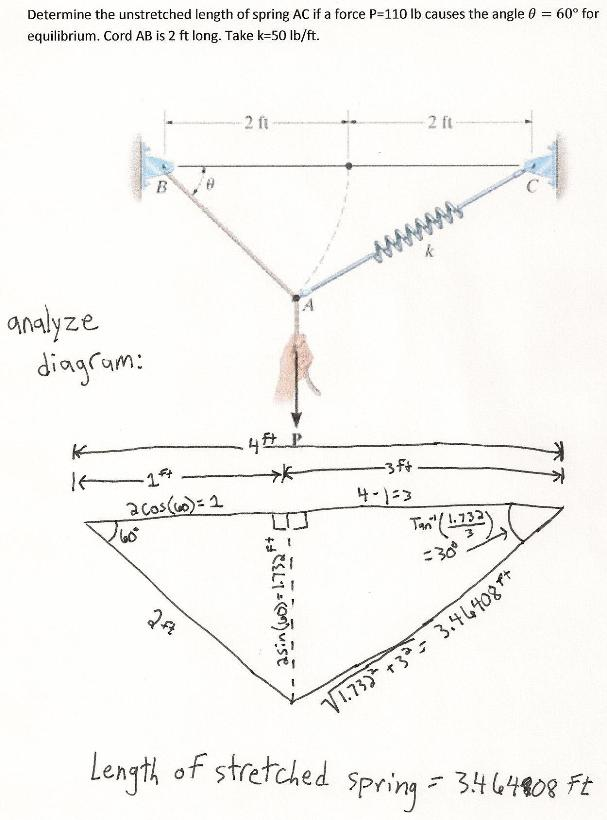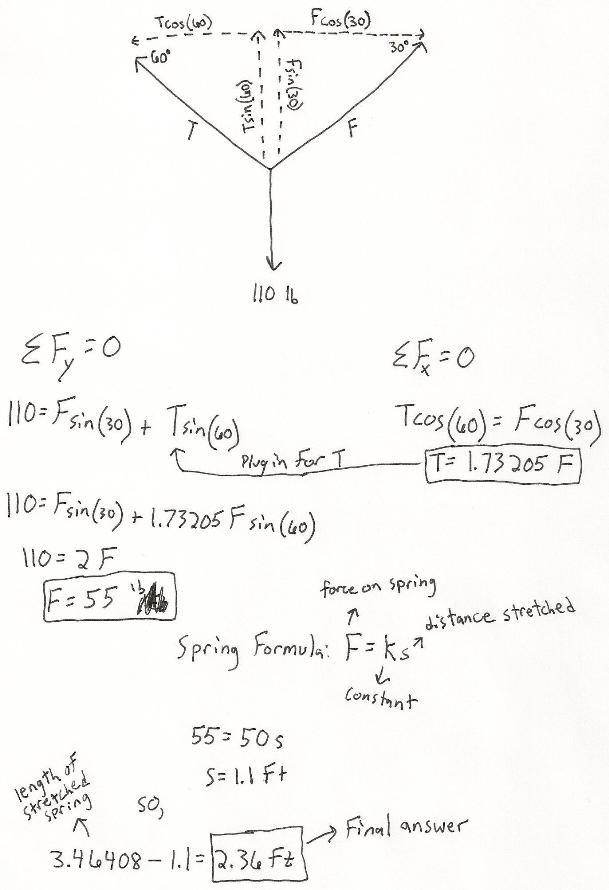statics math problems engineering equations statics basic mechanics school homework engineering math statics formulas statics problems statics problem solutions to statics problems full solution statics engineering statics problem solution statics math problems engineering equations statics basic mechanics school homework engineering math statics formulas statics problems statics problem solutions to statics problems full solution statics engineering statics problem solution
statics math problems engineering equations statics basic mechanics school homework engineering math statics formulas statics problems statics problem solutions to statics problems full solution statics engineering statics problem solution statics math problems engineering equations statics basic mechanics school homework engineering math statics formulas statics problems statics problem solutions to statics problems full solution statics engineering statics problem solution